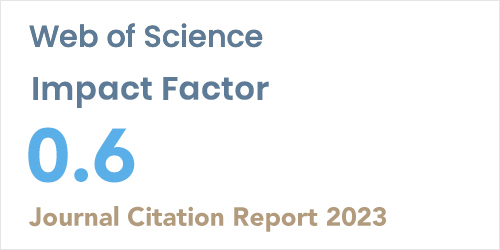ISSN: 1304-7191 | E-ISSN: 1304-7205
Volume: 41 Issue: 4 Year: 2023On the relation between categories of (m, n)-ary hypermodules and (m, n)-ary modules
1Department of Mathematics, Payamnour University, Tehran-IRAN
2School of Mathematics, University of Tehran, Tehran-IRAN
Sigma J Eng Nat Sci 2018; 9(): 85-99

#### Abstract

We introduce the category of R(m, n)-hypermodules over a Krasner (m, n)-hyperring R and obtain some categorical objects in this category such as product and coproduct. We apply the fundamental relations * and * on, M and, R respectively to construct fundamental functor from the category of R(m, n)-hypermodules into category of R/*-modules. In particular we consider the fundamental relation on (m, n)-hypermodules, and construct functor from the category of (m, n)-hypermodules to the category of (m, n)-modules. Then, we find the relations between hom, product, coproduct and fundamental functor.